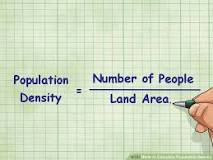Question

A

B

C

D

# 200 persons per sq. km.

Easy

## SolutionVerified by Toppr
Correct option is
D

# 200 persons per sq. km.

## To calculate the population density, you will divide the population by the size of the area. Thus, Population Density = Number of People/Land Area. The unit of land area should be square miles or square kilometers. You can use square feet or meters if you are finding the density of a smallish space.Therefore 50 lakh divided by 25000sq km equal to 200 persons per sq.km00